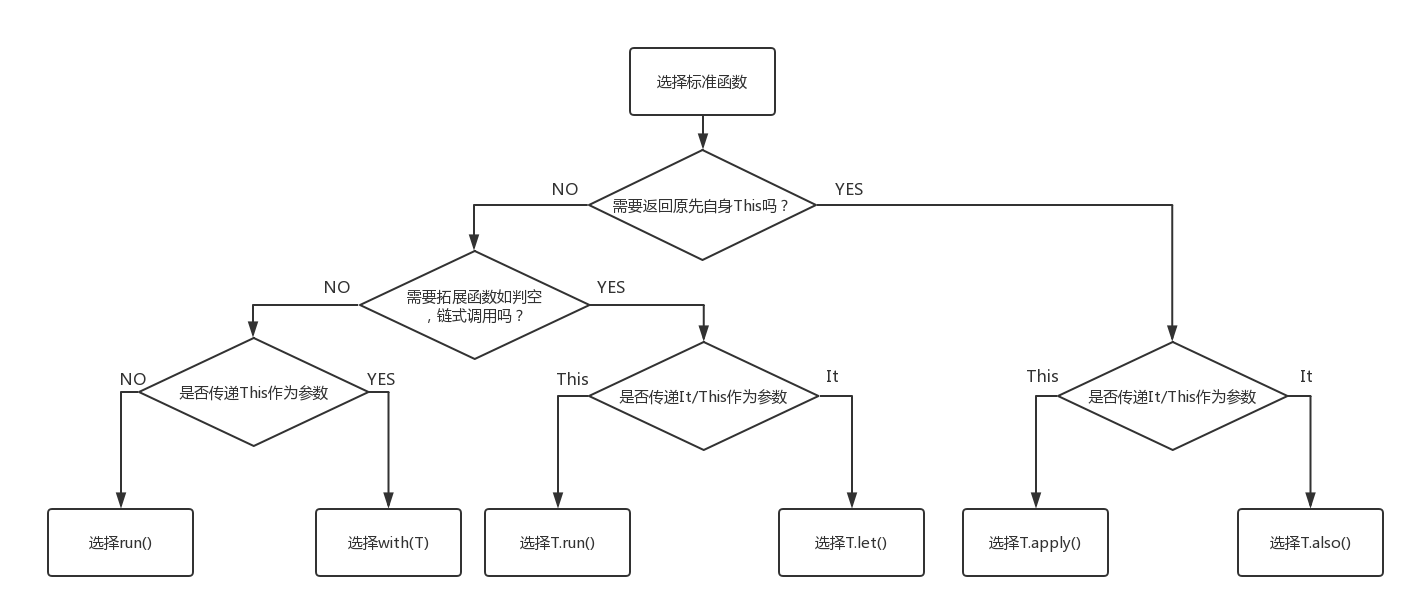# (译)掌握Kotlin的标准函数

### 范围函数

``````fun test() {
var mood = "I am sad"

run {
val mood = "I am happy"
println(mood) //I am happy
}

}
``````

``````run {
if (firstTimeView) introView else normalView
}.show ()
``````

### 范围函数的三个特性

#### 1.普通函数 VS 拓展函数

``````with(webView.setttings) {
javaScriptEnabled = true
databaseEnabled = true
}

webView.setttings.run {
javaScriptEnabled = true
databaseEnabled = true
}
``````

``````with(webView.setttings) {
this?.javaScriptEnabled = true
this?.databaseEnabled = true
}

webView.setttings?.run {
javaScriptEnabled = true
databaseEnabled = true
}
``````

#### 2.This VS it 参数

``````stringVariable?.run {
println("The length of this String is \$length")
}

stringVariable?.let {
println("The length of this String is {it.length}")
}
``````

• T.let提供了一个明显的分界线，在使用变量函数/成员的同时和外部的函数/成员划分了清晰的界限，比如你可以清楚地分辨出哪个是外部的成员，哪个是T.let中的成员
• 在this不能被省略的情况下，比如它在函数内传递了自己作为参数，”it”写起来比”this”更短且更清晰明了
• T.let允许你更好地去重命名已经存在的变量，也就是你可以把it转换成别的名字
``````stringVariable?.let {
notNullString ->
println("The length of this String is \$notNullString")
}
``````

### 3.返回当前类型 VS 返回其它类型

``````stringVariable?.let {
println("The length of this String is {it.length}")
}

stringVariable?.also {
println("The length of this String is {it.length}")
}
``````

``````val original = "abc"

// 改变当前值并传递到下一个链式函数中
original.let {

println("The original String is \$it") //"abc"

it.reverse() //改变it的变量，并把它作为参数传递到下一个let。it.reverse返回一个全新变量

}.let {

println("The reverse String is \$it") //"cba"

it.length //我们还可以改变返回it类型

}.let {

println("The length of the String is \$it") //3

}

val original = "abc"

original.also {

println("The original String is \$it") //"abc"

it.reverse() //即使我们改变返回的变量，返回的变量依旧不变。it.reverse返回一个全新变量

}.also {

println("The reverse String is \${it}") //"abc"

it.length //即使我们改变了返回类型，返回的变量依旧不变

}.also {

println("The length of the String is \$it") //"abc"

}

//如果我们要达到T.let的效果
original.also {

println("The original String is \$it") //"abc"

}.also {

println("The reverse String is \${it.reverse()}") //"cba"

}.also {

println("The length of the String is \${it.length}") //3

}
``````

``````original.also {
println("The original String is \$it") //"abc"
println("The reverse String is \${it.reverse()}") //"cba"
println("The length of the String is \${it.length}") //3
}
``````

• 它可以给同一个对象提供一个清晰的分离过程，比如实现一些小的功能块
• 在被使用之前，它可以实现十分有用的自我操作，比如实现一个链式建造过程

``````data class Data (
var id: Int,
var name: String
)

fun test() {
var data = Data(2, "Ming")
data.also {
data.name = "Hong"
}.also {
println(it) //data: id = 2, name = "Hong"
}
}
``````

``````//通常操作
fun makeDir(path: String): File {
val result = File(path)
result.mkdirs()
return result
}

//进阶操作
fun makeDir(path: String) = path.let{ File(it) }.also{ it.mkdirs() }
``````

### 回顾所有的特性

• 它是一个拓展函数
• 它传递this为它的参数
• 它返回的对象是this

``````//通常操作
fun createInstance(args: Bundle) : MyFragment {
val fragment = MyFragment()
fragment.arguments = args
return fragment
}
//进阶操作
fun createInstance(args: Bundle) = MyFragment().apply( arguments = args)
``````

``````//正常操作
fun createIntent(intentData: String, intentAction: String): Intent {
val intent = Intent()
intent.action = intentAction
intent.data = Uri.parse(intentData)
return intent
}
//进阶操作
fun createIntent(intentData: String, intentAction: String) =
Intent().apply( action = intentAction ).apply{ data = Uri.parse(intentData) }
``````

### 函数选择